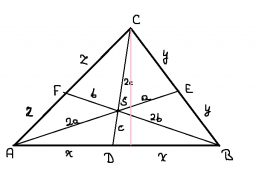# Coordinates 32183

The triangle ABC is given in the plane. A (-3,5), B (2,3), C (-1, -2) write the coordinates of the vectors u, v, w if u = AB, v = AC, w = BC. Enter the coordinates of the centers of the lines SAB (..), SAC (...), SBC (. ..)

u0 =  5
u1 =  -2
v0 =  2
v1 =  -7
w0 =  -3
w1 =  -5
x0 =  -0.5
y0 =  4
x1 =  -2
y1 =  1.5
x2 =  0.5
y2 =  0.5

### Step-by-step explanation:Did you find an error or inaccuracy? Feel free to write us. Thank you!

Tips for related online calculators
Looking for help with calculating arithmetic mean?
Line slope calculator is helpful for basic calculations in analytic geometry. The coordinates of two points in the plane calculate slope, normal and parametric line equation(s), slope, directional angle, direction vector, the length of the segment, intersections of the coordinate axes, etc.
Looking for a statistical calculator?
Our vector sum calculator can add two vectors given by their magnitudes and by included angle.